## Display the hidden estimate for the reference category in EFFECT coding for better interpretability

Started ‎09-18-2020 by
Modified ‎03-31-2020 by
Views 4,962

In this article, I give you an example of how to improve interpretability of a machine learning model by explaining the coefficients of categorical variables in regression models. The article uses the macro %CALC_REFERENCE_CATEGORY, that can be download from my github page. More details on the macro can be found in a future SAS Communities article, "The %CALC_REFERENCE_CATEGORY macro."

In my Webinar Series "Home alone? Invest now in your data science skills, and help solve the problems of the moment and the future" (LinkedIn + Medium) you find a recording of the presentation on this topic.

The interpretation of analytics results is a key topic for many data scientists. If you can better interpret and communicate the outcome of your models, you will receive a higher acceptance of your results and your work. A good interpretation of the models also provides better understanding for the business user and consequently a better usage of the analytics results in the business process. An illustrative interpretation and explanation of the analytical models also decreases the risk of last minute misunderstandings when the model is moved into production.

# A case study: Survival analysis performed for employee headcount data

The application of the macro is illustrated with a business case study for the human resources sector. It shows how survival analysis can be used to analyse employee headcount data. In the following graph, the career for each employee is illustrated by a horizontal line. You see that some careers are quite short, others are longer and some of them extend until the end of the data collection in December 2016.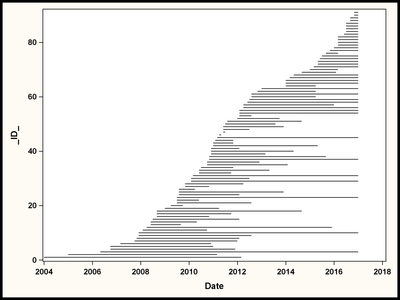You see that we are dealing with right-censored data here. To analyze such data, you can use SAS/STAT procedures like the LIFETEST, the LIFEREG or the PHREG procedure.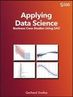This case study is taken from my SAS Press book, "Applying Data Science - Business Case Studies Using SAS." In chapters 1-4, I explain the case study "Employee Headcount Analysis." In the attachment to this article, you'll find a SAS file "CREATE_DATA_employees.sas" that creates the data from a datastep.

This example analyzes the effect of the factors DEPARTMENT, GENDER, TECHNICALKNOWHOW on the expected survival and the probability to resign within the next 6 months.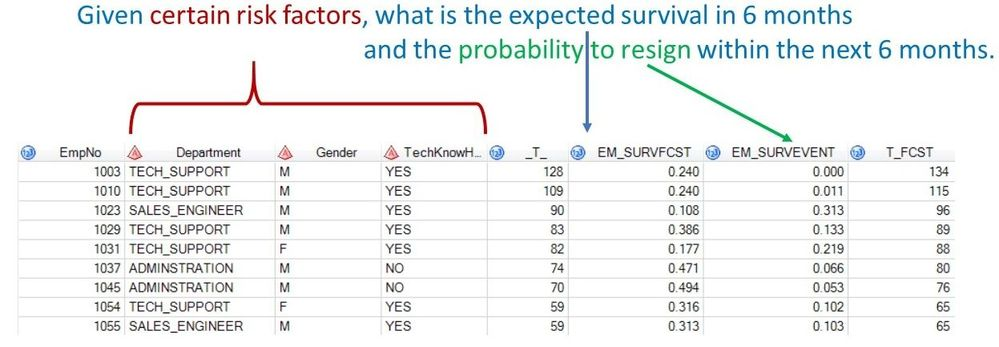# Analysis with the PHREG procedures

To perform such an analysis, you use the PHREG procedure with the following syntax.

``````proc phreg data=employees;
CLASS department gender TechKnowHow / PARAM=reference REF=first;
MODEL Duration*Status(1)= department gender TechKnowHow / SELECTION=stepwise;
run;``````

You see that a CLASS statement is used to list the categorical variables. The PARAM= option defines that the REFERENCE code shall be used here.

Note that the REF option is set to FIRST here. This specifies that the first category of each CLASS variable is used as reference category.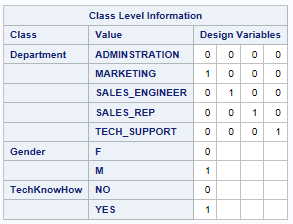The output of the PHREG procedures shows how the categorical variables are encoded by design variables. For variable DEPARTMENT, you see that the ADMINISTRATION department has been used as the reference category with a 0-value for all variables.

The interpretation of the regression coefficients for variable DEPARTMENT can now be interpreted as the difference of each department compared to the ADMINISTRATION department with value 0.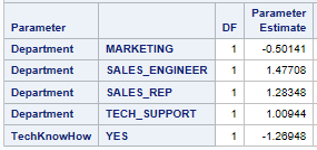You see that only the MARKETING department has a lower risk the employees resign. All other departments have a higher risk for an employee resignment.

# Defining a reference category is not always possible from a business point of view!

From a business point of view however, it is not always possible or straightforward to define a reference category to serves as the comparison basis for the other categories. And the arbitrary selection of a category is often confusing for the business user in the interpretation of the model.

We see examples where it's difficult to select a reference category in multiple business domains:

• If you analyse blood donation, it is hard to decide which blood group shall serve as the reference group.
• In credit scoring, it is often not obvious which loan type shall serve as the reference.
• In analytical CRM, it is hard to define which geographic region shall be the base region that is compared to the other regions.

# The EFFECT coding for categorical variables can help here!

The EFFECT coding also generates design variables. However, as opposed to the REFERENCE coding, the reference category gets assigned value of -1.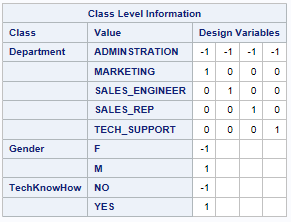The code to run such an analysis is similar to the code above. Only the value for PARAM= is changed to EFFECT.

``````proc phreg data=employees;
CLASS department gender TechKnowHow / PARAM=effect REF=first;
MODEL Duration*Status(1)= department gender TechKnowHow / SELECTION=stepwise;
run;``````

Here are the parameter estimates generated by this analysis: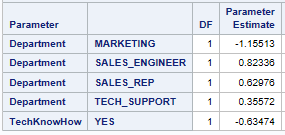You see from the parameter estimates that the coefficient for MARKETING is still the lowest value. However the values are different from the results from above when the REFERENCE coding has been used.

Note that the EFFECT coding is available for the following:

• SAS/STAT procedures: CATMOD, GENMOD, GLMSELECT, LOGISTIC, PHREG, and SURVEYPHREG
• SAS Viya Visual Statistics Procedures: GAMMOD, GAMSELECT, GENSELECT, LMIXED, LOGSELECT, MODELMATRIX, PHSELECT, PLSMOD, QTRSELECT, REGSELECT, SANDWICH, and TREESPLIT

# Graphical comparison

The following graph compares the parameter estimates from the two PHREG procedure calls graphically. The coefficients for the REFERENCE coding are shown at the right and the coefficients for EFFECT coding at the left.

Find the code for this graph in the Appendix to this plot.

You see that the distance between the individual categories is the same in the two encoding options. You also see that the values are just shifted by a constant factor.

• For the REFERENCE coding, the values are located with ADMINISTRATION = 0.
• The values for the EFFECT coding are not bound to any fixed zero value.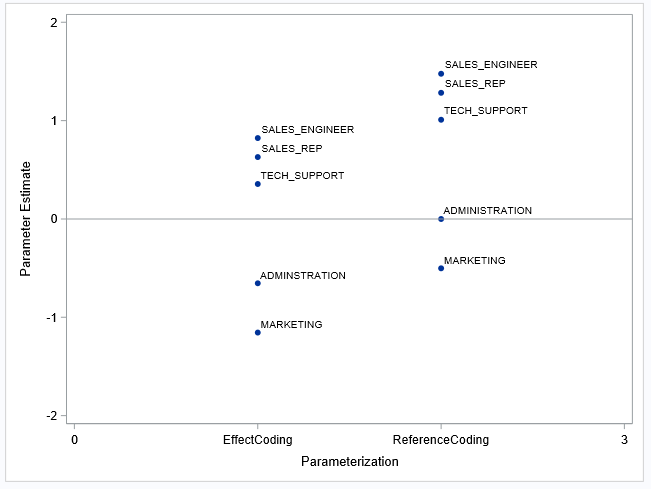From the graph you see that the (hidden) regression coefficient for ADMINISTRATION is somewhere around -0.6 or -0.7.

You see that the EFFECT coding provides regression coefficients for each category without having to artificially assign one category the zero value.

# How can you calculate the (hidden) value of the reference category in effect coding?

Of course you do not always want to look this regression coefficient up from a diagram. You want to calculate it. And this is very easy! Just:

1. Sum up the regression coefficients for the respective variable from the output of the PHREG procedure.
2. Change the sign of this sum.

In our example from above this results in the following calculation:

1. Sum it up: (-1.155)+0.823+0.630+0.356 = 0.654
2. Change the sign: -0.654

If you compare this value with the output in the graph, you see that this is a reasonable value for ADMINISTRATION.

### Warning and usage note:

Note that this calculation shall only be applied for interpretation purposes to understand the magnitude of the coefficient for the reference category. It must not be additionally included in the calculation of the predictor if new observations shall be scored. The design variables and the parameter estimates of the regression procedure output are already scaled that way that the correct prediction is made for the reference category, even if no value is shown in the parameter estimate output.

# The %CALC_REFERENCE_MACRO does the work for you!

In my SAS Press book "Applying Data Science - Business Case Studies Using SAS" a SAS macro is introduced that automatically performs this calculation for you. You can download this macro at my github page. The macro is tested for the SAS/STAT procedures:  GLMSELECT, PHREG, LOGISITC and the SAS Viya Visual Statistics procedures: LOGSELECT, GENSELECT, PHSELECT.
For the employees data example with the PHREG procedure, the macros generates the following output: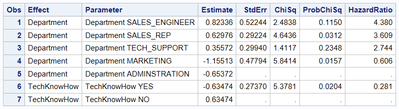Observations 5 and 7 have been inserted by the %CALC_REFERENCE_CATEGORY macro.

# Feedback from the business user

I have used the EFFECT coding in many analyses across industries and business domains where I've built regression models. Business departments usually do not appreciate the fact that they have to select a specific category as a reference. And it is often counter intuitive for them why a certain category should receive the value 0 and act as the comparison level for the other categories.

In days where the interpretation of the analytical model and the involvement of the business user play and increasing role, I often use this method to better communicate my findings and my machine learning models.

# Appendix - Code to create the comparative graph

This code shows how the graph comparing the two encodings has been created.

### 1. Prepare the coefficients from REFERENCE coding

Add a record for the reference category at the begin of the datastep. You use the OUTPUT statement here and only export this once (before the first record, _N_=1).

``````data RefParmEstimates_XT(Keep=parameter classval0 estimate ModelID);
if _N_ = 1 then do;
Parameter = "Department";
Estimate = 0;
ModelID=2;
output;
end;
set RefParmEstimates;
ModelID = 2;
if parameter = "Department";
output;
run;``````

Assign MODELID = 2 for the REFERENCE encoding.

Restrict the output only to coefficients for parameter DEPARTMENT.

### 2. Prepare the coefficients from EFFECT coding

Use the %CALC_REFERENCE_CATEGORY macro to add and calculate a record for the ADMINISTRATION department in EFFECT coding.

``%Calc_Reference_Category(ParmEst=EmpParmEstimates, ClassLevels=EmpClassLevels,PROC=PHREG);``
Run a datastep to prepare the data in the same structure as in REFERENCE coding.
``````data EffectParmEstimates;
set _parmest_xt_;
keep estimate parameter ClassVal0 ModelID;
ClassVal0 = scan(Parameter,2);
Parameter = scan(Parameter,1);
ModelID=1;
if effect = "Department";
run;``````
You assign MODELID = 1 for the EFFECT encoding.

You restrict the output only to coefficients for parameter DEPARTMENT.

### 3. Append the two datasets

You append these two datasets with a SAS datastep.
``````data ParmEstimates;
set RefParmEstimates_XT
EffectParmEstimates;
run;``````

### 4. Create the Plot with SGPLOT procedure

First a FORMAT is created for the two encodings.
``````proc format;
value mid
1="EffectCoding"
2="ReferenceCoding";
run;``````
Use the SGPLOT procedure to create the plot.
``````proc sgplot data=ParmEstimates;
format ModelID Mid.;
scatter x=ModelID y=Estimate / datalabel=ClassVal0 markerattrs=(symbol = circlefilled);
xaxis values=(0 to 3 by 1)  label="Parameterization";
yaxis min=-2 max=2;
refline 0 / axis=y;
run;``````
Note that variable CLASSVAL0 contains the category name and is used as a DATALABEL.

Use the MID format that displays the value on the x-axis.

Use the XAXIS and YAXIS statement to format the axis with the MININUM and MAXIMUM value or by providing a range of values. Note the BY 1 in `values=(0 to 3 by 1)` is important to make sure that only integer steps are shown at the x-axis.

For better visibility it is advisable to add a reference line at the y-axis a value 0. Use the REFLINE statement for that.

# Other articles in this series

Version history
Last update:
‎03-31-2020 05:30 PM
Updated by:Contributors
Article Labels
Article Tags